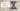# Integer to Roman conversion - Leet Code Solution

September 13, 2019## Problem Statement

Roman numerals are represented by seven different symbols: I, V, X, L, C, D and M.

``````Symbol       Value
I             1
V             5
X             10
L             50
C             100
D             500
M             1000``````

For example, two is written as II in Roman numeral, just two one’s added together. Twelve is written as, XII, which is simply X + II. The number twenty seven is written as XXVII, which is XX + V + II.

Roman numerals are usually written largest to smallest from left to right. However, the numeral for four is not IIII. Instead, the number four is written as IV. Because the one is before the five we subtract it making four. The same principle applies to the number nine, which is written as IX. There are six instances where subtraction is used:

``````I can be placed before V (5) and X (10) to make 4 and 9.
X can be placed before L (50) and C (100) to make 40 and 90.
C can be placed before D (500) and M (1000) to make 400 and 900.``````

Given an integer, convert it to a roman numeral. Input is guaranteed to be within the range from 1 to 3999.

## Solution

The question looks simple interms that we need to keep mappings. There are three special cases i.e. where subtraction is used.

Lets look at the code:

``````public String intToRoman(int num) {
Map<Integer, String> map = new HashMap();
map.put(1, "I"); map.put(5, "V"); map.put(10, "X");
map.put(50, "L"); map.put(100, "C"); map.put(500, "D"); map.put(1000, "M");
map.put(4, "IV"); map.put(9, "IX"); map.put(40, "XL"); map.put(90, "XC");
map.put(400, "CD"); map.put(900, "CM");

int[] sequence = {1000, 900, 500, 400, 100, 90, 50, 40, 10, 9, 5, 4, 1};

StringBuffer sb = new StringBuffer();
for (int i=0; i<sequence.length; i++) {
int base = sequence[i];

while (num >= base) {
sb.append(map.get(base));
num -= base;
}
}

return sb.toString();
}``````